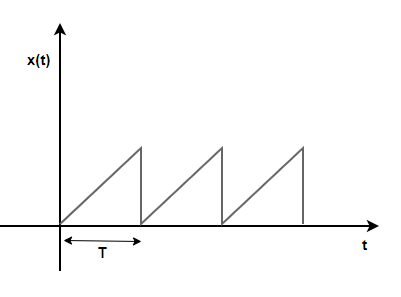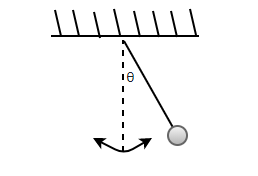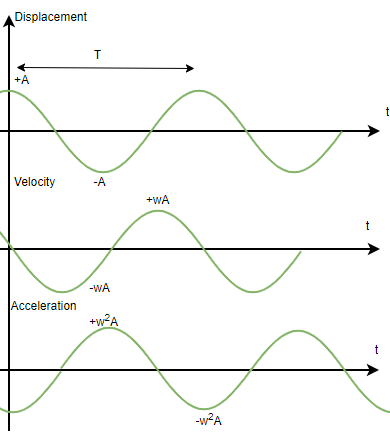GFG App
Open AppBrowser
Continue

# Velocity and Acceleration in Simple Harmonic Motion

Simple Harmonic Motion is a periodic motion that repeats itself after a certain time period. It can be seen almost everywhere in real life, for example, a body connected to spring is doing simple harmonic motion. It is essential to know the equation for the position, velocity, and acceleration of the object. These equations help us deduce information about the object from the SHM and predict its behavior. Let us look at how to extract this information from an SHM in detail.

### Periodic and Oscillatory Motion

Consider an example of an insect trying to climb up the wall, this insect climbs up to a height and then falls back down again. Notice, that the motion of this insect is repeating itself after some time. This kind of motion is called periodic motion. A periodic motion repeats itself after a certain interval of time. In the figure, some graphs are shown which represent a periodic motion. The graph has a pattern that is repeating, which means that the y-position of the object increases and then decreases periodically.Periodic and Oscillatory motions may seem the same, but they have a minor difference. Every oscillatory motion is a periodic motion but not vice-versa. Periodic motions just repeat themselves after a certain interval of time, but oscillatory motions move to and fro around a mean position. Simple Harmonic Motion is the simplest type of oscillatory motion.

### Simple Harmonic Motion

Simple Harmonic Motion is a kind of periodic motion where the object moves to and fro around its mean position. This motion arises when the force acting on the body is directly proportional to the displacement of the body from its mean position. The time period, in this case, remains constant. The time period is denoted by “T” and the distance of the mean position from the extreme position is called amplitude, it is denoted by A. An oscillating pendulum is an example of Simple Harmonic Motion.The general equation for the displacement(x) of the object at any particular time is given by,Here,anddenotes the phase shift.

Similarly, the equation for the velocity of the object in SHM can be found by differentiating this equation.Then, the equation for acceleration becomes,Notice in the figure above, that all three values displacement, velocity, and acceleration in SHM have the same time period as SHM, but they have a phase of 90° between each of them.

### Sample Problems

Question 1: The equation for the SHM is given below.

x(t) = 5cos(2t)

Calculate the maximum acceleration and velocity.

General equation of SHM is given by,In this case, A = 5,Maximum velocity will be,⇒ v = (5)(2)

⇒ v = 10 m/s

Maximum acceleration will be,⇒ a = – (5)(2)2

⇒ a = -20 m/s2

Question 2: The equation for the SHM is given below.

x(t) = 10sin(5t)

Calculate the maximum acceleration and velocity.

General equation of SHM is given by,In this case, A = 10,Maximum velocity will be,⇒ v = (10)(5)

⇒ v = 50 m/s

Maximum acceleration will be,⇒ a = – (10)(5)2

⇒ a = -250 m/s2

Question 3: The equation for the SHM is given below.

x(t) = 100sin(12t)

Calculate the time and velocity at t =General equation of SHM is given by,In this case, A = 100,We know,Plugging the values into the equation,⇒Equation for velocity will be found by differentiating the given equation,

x(t) = 100sin(12t)⇒At t =Question 4: The equation for the SHM is given below.

x(t) = 100sin(2t + 5)

Calculate the time and velocity at t =General equation of SHM is given by,In this case, A = 100,We know,Plugging the values into the equation,⇒Equation for velocity will be found by differentiating the given equation,

x(t) = 100sin(2t + 5)⇒At t =Question 5: The equation for the SHM is given below.

x(t) = 2sin(t – 5)

Calculate the time-period, acceleration and velocity at t =General equation of SHM is given by,In this case, A = 2,We know,Plugging the values into the equation,⇒Equations for velocity and accelerations will be found by differentiating the given equation,

x(t) = 2sin(t – 5)⇒At t =⇒ a(t) = -2sin(t – 5)

At t =My Personal Notes arrow_drop_up